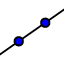# UNIT 2 LESSON 4 GRAPH A LINE IN POINT-SLOPE FORM

What is the slope of the line ?

Select all that apply
• A
• B
• C
• D

What is the given point?

Select all that apply
• A
• B
• C
• D

## GRAPHING A LINE FROM POINT SLOPE FORM

Graph a line clicktwice. To plot the point find where you want to put the point on the graph and click on the point then use the slope to plot an additional point. Click the check box to check your work.

## GRAPHING A REAL-WORLD LINEAR MODEL

Ivan's sister agrees to loan him some money if he agrees to repay \$2 every week. The equation models the amount of the loan remaining, where x is time (in weeks) and y is the amount left on the loan (in dollars). What are the slope and the point the equation gives you? Graph the equation below.

What amount was the loan for?

After how many weeks will he have the paid off the loan?